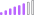cancel
Showing results for
Did you mean:Post Patron

## A circular dependency was detected HELP!

Hello,

Would like to ask for your help. Im trying to get the total of two columns but when im creating a total column it shows an error of:

Total ColumnC_Contractor_Assigned_FIELD + C_Contractor_Assigned_MANUAL

Error: A circular dependency was detected: ED[C_Contractor_Assigned_FIELD], ED[C_Contractor_Assigned_Total], ED[C_Contractor_Assigned_FIELD].

Here are my two columns to be added:

Im trying to add column A =

C_Contractor_Assigned_FIELD = CALCULATE(DISTINCTCOUNT(ED[ID]),ED[IN_FIELD] = "YES"))
and column B = C_Contractor_Assigned_MANUAL = CALCULATE(DISTINCTCOUNT(ED[ID]),FILTER(ED,ED[VENDOR_ASSIGNED] = "YES" && ED[IN_FIELD] = "NO"))

Thank you!

1 ACCEPTED SOLUTIONPost Patron

Hi Everyone!

Solved already. I just used the quick measure in powerbi. It just worked! 🙂

3 REPLIES 3Super User

@icdns , I saw equal to in formula. I am not sure is it typo here. it is like that in formula

these should be like

column A = C_Contractor_Assigned_FIELD - CALCULATE(DISTINCTCOUNT(ED[ID]),ED[IN_FIELD] = "YES"))

column B = C_Contractor_Assigned_MANUAL - CALCULATE(DISTINCTCOUNT(ED[ID]),FILTER(ED,ED[VENDOR_ASSIGNED] = "YES" && ED[IN_FIELD] = "NO"))

These seem fine. Can you share sample pbix after removing sensitive data.

refer :

column A = C_Contractor_Assigned_FIELD - CALCULATE(DISTINCTCOUNT(ED[ID]),ED[IN_FIELD] = "YES"))

column B = C_Contractor_Assigned_MANUAL - CALCULATE(DISTINCTCOUNT(ED[ID]),FILTER(ED,ED[VENDOR_ASSIGNED] = "YES" && ED[IN_FIELD] = "NO"))Post Patron

Hello @

Sorry for the confusion but it's:

C_Contractor_Assigned_FIELD = CALCULATE(DISTINCTCOUNT(ED[ID]),ED[IN_FIELD] = "YES"))

C_Contractor_Assigned_MANUAL = CALCULATE(DISTINCTCOUNT(ED[ID]),FILTER(ED,ED[VENDOR_ASSIGNED] = "YES" && ED[IN_FIELD] = "NO"))

TOTAL = C_Contractor_Assigned_FIELD  + C_Contractor_Assigned_MANUAL

But when i tried making the total column it shows an circular dependency error.Post Patron

Hi Everyone!

Solved already. I just used the quick measure in powerbi. It just worked! 🙂